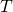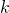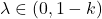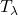# Approximating fixed points of demicontractive mappings via the quasi-nonexpansive case

### Full PDFcarpathian_2023_39_1_73_84

We prove that the convergence theorems for Mann iteration used for approximation of the fixed points of demicontractive mappings in Hilbert spaces can be derived from the corresponding convergence theorems in the class of quasi-nonexpansive mappings. Our derivation is based on an important auxiliary lemma (Lemma 3.2), which shows that ifis-demicontractive, then for any,is quasi-nonexpansive.

In this way we obtain a unifying technique of proof for various well known results in the fixed point theory of demicontractive mappings.

We illustrate this reduction technique for the case of two classical convergence results in the class of demicontractive mappings: [Măruşter, Șt. The solution by iteration of nonlinear equations in Hilbert spaces.  Proc. Amer. Math. Soc. 63 (1977), no. 1, 69–73] and [Hicks, T. L.; Kubicek, J. D. On the Mann iteration process in a Hilbert space. J. Math. Anal. Appl. 59 (1977), no. 3, 498–504].